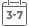# Introduction to Random Processes in Engineering

• Publish Date: 2005-06-09
• Binding: Paperback
• Author: A. V. Balakrishnan
• Sale
• \$16.46
• Regular price \$119.77Breaking with the traditional treatment of random processes in engineering

On the surface, Introduction to Random Processes in Engineering is simply a first-rate textbook for senior or first-year graduate engineering courses in stochastic processes. A closer look, however, reveals an innovative book-rich with examples and commonsense explanations-that demystifies theories, eliminates ambiguities, and provides a solid, up-to-date introduction to this important subject.

Departing from the classical texts of the sixties and seventies in its coverage of random signals and data processing, Introduction to Random Processes in Engineering addresses the latest advances in communication, control engineering, and signal processing by allowing all processes to be multidimensional with an emphasis on discrete-time processes and systems.

Unlike current texts, this volume provides a strong mathematical perspective for its engineering topics without getting bogged down in technicalities. It employs mathematics to achieve clarity and precision, and at times even uses the theorem/proof style to emphasize mathematical fine points. This approach is particularly advantageous when dealing with random data, and when building an understanding of the many computer programs routinely used, its theoretical principles, and the results it generates.

Assuming a senior-level background in probability theory and some acquaintance with linear systems and signals, the book provides:
* A review chapter of the formulas used later in the book
* Illustrative examples
* Emphasis in simulation techniques
* Problems accompanying each chapter that often introduce the student to other relevant material
* Notes and comments following each chapter that encourage additional reading as well as historical explorations in the field
* Tips for using the material at various levels of instruction

With its logical and systematically ordered presentation of the material, as well as its fresh approach, Introduction to Random Processes in Engineering is both a superior textbook and a valuable reference for practicing engineers and researchers in the field.

MORE FROM THIS COLLECTION